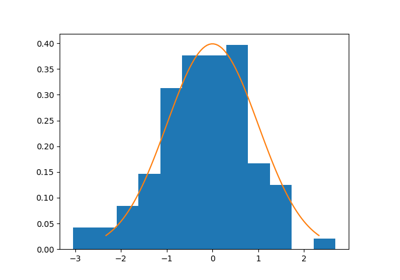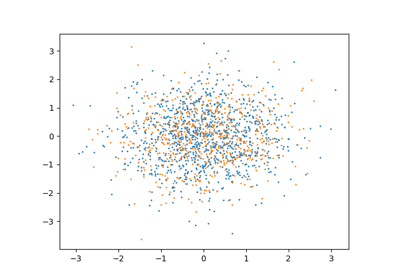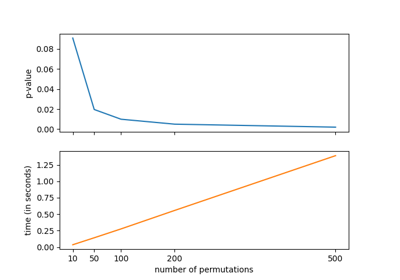# HypothesisTest#

class HypothesisTest(pvalue, statistic)[source]#

Class containing the results of an hypothesis test.

Methods

 `__init__`(pvalue, statistic)
Parameters
• pvalue (float) –

• statistic (T) –

__init__(pvalue, statistic)#
Parameters
• pvalue (float) –

• statistic (T) –

Return type

None

## Examples using `dcor.HypothesisTest`#The distance correlation t-test of independence

The distance correlation t-test of independenceThe energy distance test of homogeneity

The energy distance test of homogeneityThe distance covariance test of independence

The distance covariance test of independence